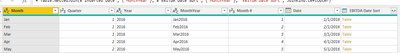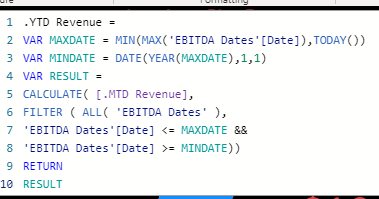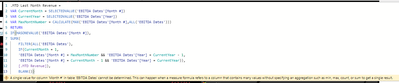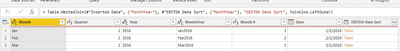cancel
Showing results for
Did you mean:Frequent Visitor

## Creating Y-o-Y % Change using DAX

I am trying to create a year over year % change using DAX but the issue I am encoutering is my date table is not the traditional date table due to how the excel file extracts information. I have DAX writtien to get MTD revenue already, just need to determine how to get a measure for previous year revenue. The screenshots below in order are 1) modeling, 2) DAX for my MTD Revenue measure, 3)how my date table is set up along with the formatting. Any help would be greatly appreciated!4 REPLIES 4Super User

Hi mjr:

Are you able to add a Date Table? If so, you can mark as Date Table and connect the Date field to your Financials date field. I'll paste DAX date table code below. You then go to New Table and put this code in.

I'd start off with just a revenue calculation, which may be the mtd measure you have? I think you just need to change your measures name from mtd Revenue to "Revenue"

Then

LY Revenue = CALCULATE([Revenue], DATEADD(Dates[Date], -1, YEAR))

YoY Revenue = [Revenue] - [LY Revenue]

YoY Rev % = DIVIDE([YoY Revenue], [LY Revenue])

If I'm off track, can you share sample data?

Thanks and hope this helps..

Here is DAX Date Table. * Critical to mark as Date table, validating on Date field.

Dates =

-- Specify a start date and end date

VAR StartDate = Date(2021,1,1)

VAR EndDate = Today() + 243

VAR FiscalMonthEnd = 12

-- Generate a base table of dates

VAR BaseTable = Calendar(StartDate, EndDate)

-- Add the Year for each individual date

-- Add the calendar month and other month related data for each date

Years,

"Month",MONTH([Date]),

"Year and Month Number",FORMAT([Date],"YYYY-MM"),

"Year and Month Name",FORMAT([Date],"YYYY-MMM"),

"Fiscal Year", IF( FiscalMonthEnd = 12, YEAR([Date]), IF( MONTH([DATE]) <= FiscalMonthEnd, YEAR([DATE])-1, YEAR([Date]))),

"Fiscal Month", IF( FiscalMonthEnd = 12, MONTH([Date]),

IF( MONTH([Date]) <= FiscalMonthEnd, FiscalMonthEnd + MONTH([Date]), MONTH([Date]) - FiscalMonthEnd))

)

-- Add the Quarter and other quarter related data for each date

Months,

"Quarter",ROUNDUP(MONTH([Date])/3,0),

"Year and Quarter",[Year] & "-Q" & ROUNDUP(MONTH([Date])/3,0))

-- Add the Day and other day related data for each date

Quarters,

"Day",DAY([Date]),

"Day Name",FORMAT([Date],"DDDD"),

"Day Of Week",WEEKDAY([Date]),

"Day Of Year", DATEDIFF (DATE(YEAR([Date]),1,1), [Date], DAY) + 1)

-- Add the Week (assuming each week starts on a Sunday) and other week related data for each date

Days,

"Week Of Month (Sunday)",INT((DAY([Date])-1)/7)+1,

"Week of Year (Sunday)",WEEKNUM([Date],1),

"Year and Week (Sunday)",[Year] & "-W" & WEEKNUM([Date],1))

-- Add an 'Is Working Day' column which will be true for all days but Saturday and Sunday.

Weeks,

"Is Working Day", NOT WEEKDAY( [Date] ) IN {1,7})

RETURN WorkingDaysFrequent Visitor

Date table is not an option, which is why this is more complicated. I was thinking of using my YTD Revenue measure but not sure how to set the min date.Super User

Hi:

I believe you can use this pattern for MTD LY. You would substitute your table name(with year and month no) for Calendar as used below. You will see the use of taking 1 away from year variable to get to LY. I hope this helps..

Previous Month Sales =
VAR CurrentMonth = SELECTEDVALUE( 'Calendar'[Fin Month Number] )
VAR CurrentYear = SELECTEDVALUE( 'Calendar'[Fin Year] )
VAR MaxMonthNum = CALCULATE( MAX( 'Calendar'[Fin Month Number] ), ALL( 'Calendar' ) )

RETURN
IF( HASONEVALUE( 'Calendar'[Fin Month Number] ),
SUMX(
FILTER( ALL( 'Calendar' ),
IF( CurrentMonth = 1,
'Calendar'[Fin Month Number] = MaxMonthNum && 'Calendar'[Fin Year] = CurrentYear - 1,
'Calendar'[Fin Month Number] = CurrentMonth - 1 && 'Calendar'[Fin Year] = CurrentYear )),
[Total Sales] ),
BLANK() )Frequent Visitor

I had the following error saying a single Month # cannot be determined but I am confused how this could be the case? The screenshots below are as follows... 1) The measure I am attempting (and failing) 2) what my "Date Table" looks like and 3) the sorting table you see in the last column of the second one.